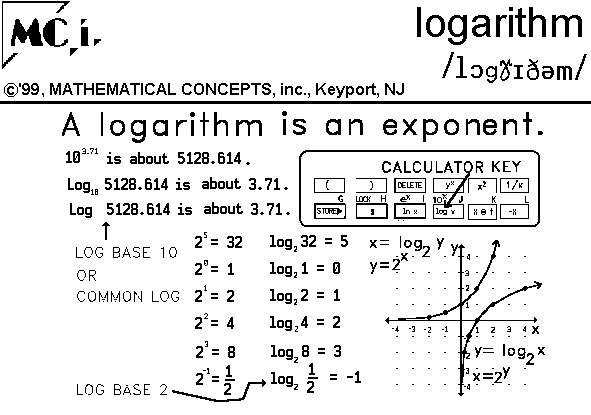IN MATH: 1. symbol for logarithm. EX..

 See: Laws/Rules of Logrithmic Computation Use of log-log plane and semi-log plane log-log plane, no axes semi-log plane, no axes Exponential Function, exp(x) or ex Lograthmic Functions, logb(x) Natual Log Function, ln(x) or loge(x) HOW TO APPRECIATE LOGS WITHOUT FEELING OUT ON A LIMB - xa(xb) etc., writing log equation from exponential equation VOCABULARY- Function and Relation Library - Many functions w/their features & graphs

IN ENGLISH: 1. n. a dead piece of wood, usually the trunk of a tree or a large branch usually without leaves or smaller branches. EX. We burn logs in the fireplace.IN MATH: 1. n. an exponent. EX. Write a log equation to solve.

IN ENGLISH: 1. as defined above.

Simplify: 1. log 1000 2.3.IN MATH: 1. n. rule which returns for each arguement the exponent to which the base must be raised in order to get the arguement; the inverse of the exponential function.

APPLICATION: (see list 340)